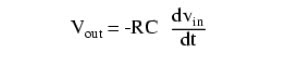## Monday, November 16, 2009

### Rangkaian Diferensiator Op-Amp

Diferensiator circuit functions to produce a voltage which is a function of the time differential input voltage. Diferensiator circuit is basically a pass-filter for condenser consisting of rows and row resistor. Because the condenser reactance increases if the frequency falls, this circuit eliminates the low frequency components of an input. If there is input level applied to diferensiator, voltage on the condenser is changed in an instant so that there is voltage on the resistor decreases exponentially in accordance with the formulaCR is a constant time, ie the product of the resistance capacity. In one time constant, voltage condenser membentangi approximately 63%. It takes nearly 5 time constants to empty the condenser.Applications for this, besides representing the derivative calculus function inside of an analog computer, include rate-of-change indicators for process instrumentation. One such rate-of-change signal application might be for monitoring (or controlling) the rate of temperature change in a furnace, where too high or too low of a temperature rise rate could be detrimental. The DC voltage produced by the diferensiator circuit could be used to drive a comparator, which would signal an alarm or activate a control if the rate of change exceeded a pre-set level.

Skema Rangkaian Elektronika